#### Linear filters

In the context of signal processing, a filter is a transformation that maps one signal to another. Each signal is a function of time, and the filter is like a black box that receives the one signal as input, and produces another as output. If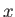represents an entire signal (over all times), then let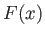represent the resulting signal after running it through the filter.

A linear filter is a special kind of filter that satisfies two algebraic properties. The first algebraic property is additivity, which means that if two signals are added and sent through the filter, the result should be the same as if they were each sent through the filter independently, and then the resulting transformed signals were added. Using notation, this is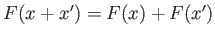for any two signalsand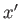. For example, if two different sounds are sent into the filter, the result should be the same whether they are combined before or after the filtering. This concept will become useful as multiple sinusoids are sent through the filter.

The second algebraic property is homogeneity, which means that if the signal is scaled by a constant factor before being sent though the filter, the result would be the same as if it were scaled by the same factor afterwards. Using notation, this means that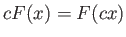for every constant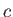and signal. For example, this means that if we double the sound amplitude, then the output sound from the filter doubles its amplitude as well.

A linear filter generally takes the form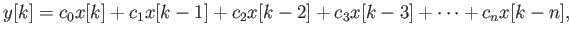(11.3)

in which each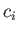is a constant, and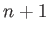is the number of samples involved in the filter. One may consider the case in which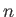tends to infinity, but it will not be pursued here. Not surprisingly, (11.3) is a linear equation. This particular form is a causal filter because the samples on the left occur no later than the sample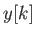. A non-causal filter would require dependency on future samples, which is reasonable for a recorded signal, but not for live sampling (the future is unpredictable!).

Here are some examples of linear filters (special cases of (11.3)). This one takes a moving average of the last three samples: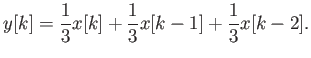(11.4)

Alternatively, this is an example of exponential smoothing (also called exponentially weighted moving average):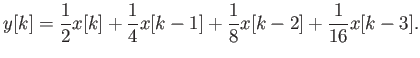(11.5)

Steven M LaValle 2020-11-11